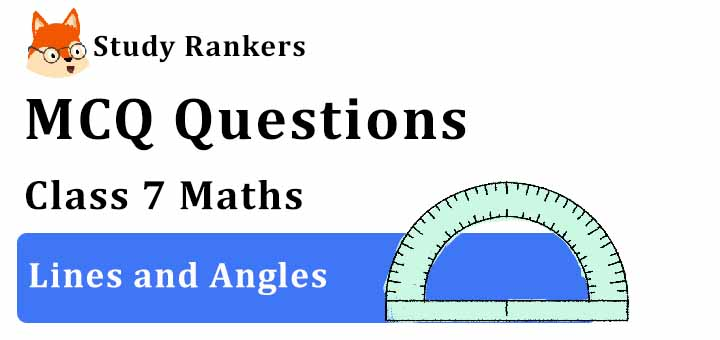## MCQ Questions for Class 7 Maths: Ch 5 Lines and Angles1. A line that intersects two or more lines at distinct points is called
(a) parallel
(b) transversal
(c) intersecting
(d) None of these
► (b) transversal

2. What happens to the measurement of an angle after the extension of its arms?
(a) Doubles
(b) Triples
(c) Remains the same
(d) Cannot be said
► (c) Remains the same

3. Find the angle, which is equal to its supplement.
(a) 60°
(b) 90°
(c) 120°
(d) 30°
► (b) 90°

4. What is the supplement of 105°
(a) 65°
(b) 75°
(c) 85°
(d) 95°
► (b) 75°

5. What do we call an angle whose measurement is exactly equal to 0o?
(a) An obtuse angle
(b) A straight angle
(c) A zero angle
(d) A right angle
► (c) A zero angle

6. Identify which of the following pairs of angles are complementary.
(a) 65°, 115°
(b) 130°, 50°
(c) 63°, 27°
(d) 112°, 68°
► (c) 63°, 27°

7. If two lines intersect at a point, then the vertically opposite angles are always ________
(a) supplementary
(b) equal
(c) unequal
(d) none of these
► (b) equal

8. What are the lines which lie on the same plane and do not intersect at any point called?
(a) Perpendicular lines
(b) Intersecting lines
(c) Parallel lines
(d) Collinear lines
► (c) Parallel lines

9. If two angles are supplementary then the sum of their measures is __________.
(a) 45°
(b) 180°
(c) 90°
(d) 360°
► (b) 180°

10. Find the angle, which is equal to its complement.
(a) 45°
(b) 25°
(c) 35°
(d) 30°
► (a) 45°

11. If two adjacent angles are supplementary, then they form _________ .
(a) a linear pair of angles
(b) vertically opposite angles
(c) Corresponding angles
(d) a ray
► (a) a linear pair of angles

12. What is the measure of the complement of 65°?
(a) 25°
(b) 55°
(c) 65°
(d) 45°
► (a) 25°

13. What is the measure of the complement of 41°?
(a) 135°
(b) 15°
(c) 49°
(d) None of these
► (c) 49°

14. Which instrument is used to measure or construct angles?
(a) Compasses
(b) Scale
(c) Protractor
(d) Set squares
► (c) Protractor

15. The sum of all angles around a point is
(a) 0deg
(b) 180deg
(c) 360deg
(d) 90deg
► (c) 360deg

16. How many rays can be drawn from a given point?
(a) 2
(b) 5
(c) 8
(d) Infinitely many
► (d) Infinitely many

17. What do we call an angle which exactly measures 90°?
(a) An obtuse angle
(b) An acute angle
(c) A right angle
(d) A reflex angle
►

18. Identify which of the following pairs of angles are supplementary.
(a) 45°, 45°
(b) 63°, 27°
(c) 112°, 68°
(d) 80°, 10°
► (c) 112°, 68°